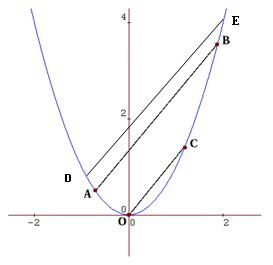#### You may also like### Power Up

Show without recourse to any calculating aid that 7^{1/2} + 7^{1/3} + 7^{1/4} < 7 and 4^{1/2} + 4^{1/3} + 4^{1/4} > 4 . Sketch the graph of f(x) = x^{1/2} + x^{1/3} + x^{1/4} -x### Snookered

In a snooker game the brown ball was on the lip of the pocket but it could not be hit directly as the black ball was in the way. How could it be potted by playing the white ball off a cushion?### Climbing

Sketch the graphs of y = sin x and y = tan x and some straight lines. Prove some inequalities.

# Parabella

##### Age 16 to 18 Challenge Level:

Thank you to all the following students who sent in very good solutions to this problem: Alex from Stoke-on-Trent Sixth Form College, Dapeng Wang from Claremont Fan Court School, Manuele Cavalli-Sforza from the British School of Manila, Jamie Beacom from Omagh Academy, Feline Angel from Wootton Upper School and Biren from The Heathland School. Well done all of you.

Biren approached it like this:

The question asks you to prove that $a+b=c$.

You already know that the two lines are parallel.This tells us that they have the same gradient.

To work out the gradients we should try to imagine we draw a right angled triangle under both lines.

We then divide the change in the $y$ direction by the change in the $x$ direction.

The change in the $x$ direction of the line $AB$ is equal to $b-a$ (small letters refer to the co-ordinates).

The change in the $y$ direction is equal to $$b^2 - a^2$$(these letters also refer to the co-ordinates).

Thus the gradient of line $AB$ is equal to: $$\frac {b^2 - a^2}{b - a} = \frac {(b-a)(b+a) }{ b-a} = b+a$$ The change in the $y$ direction of the line $OC$ is $c^2$ The change in the $x$ direction is equal to $c$. Thus the gradient of the line $OC$ is $$\frac {c^2}{c} = c$$

We know that both lines, $AB$ and $OC$ are parallel, and so they must have the same gradient.

So $b+a = c$Alex extended the result as follows: Let $d$ and $e$ be the $x$ coordinates of D and E respectively. As OC is parallel to DE, the relationship of OC to DE is the same as it was to AB, therefore $c=d+e$ just as $c=a+b$. The $x$ coordinates of the midpoints of AB, DE and OC and all the same, because $$\frac{(a+b)}{2 }= \frac{(d+e)}{2} = \frac{c}{2}.$$ The straight line $x = \frac {c}{2}$ passes through the midpoints of all the parallel lines.

Others approached the problem in a slightly different way:

Let $y = mx + n$ be the equation of the line going through the points $A(a, a^2 )$ and $B(b, b^2 )$.

At $A$, $a^2 = ma + n$

At $B$, $b^2 = mb + n$

From this: $$a^2 - b^2 = ma - mb = m (a - b).$$ Dividing by $(a-b)$: $$m = \frac {a^2 - b^2}{a - b} = \frac {(a + b)(a - b)}{a - b} = a + b$$ The line $OC$ passes through the origin and the point $C(c, c^2 )$.

Because it passes through the origin, its equation is of the form: $$y = ux$$ Because it is parallel to the line passing through $A$ and $B$, $u = m$, so its equation is: $$y = (a + b)x$$ Since it passes through $C$: $$c^2 = (a + b)c$$ or: $$c = a + b.$$